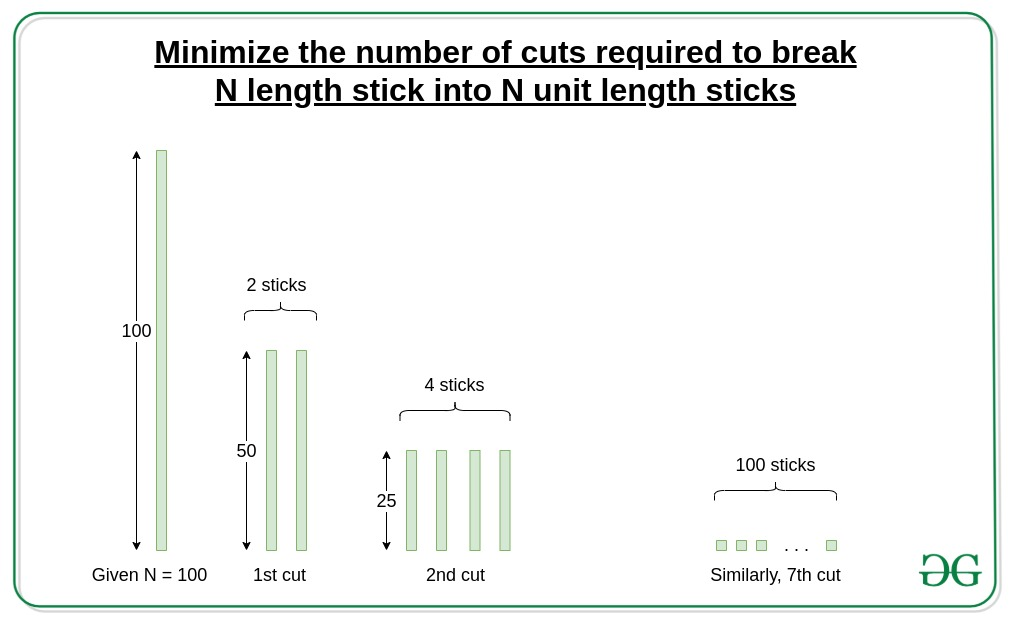Minimize number of cuts required to break N length stick into N unit length sticks

• Difficulty Level : Easy
• Last Updated : 31 Mar, 2021

Given an integer N denoting the length of a given stick, the task is to minimize the time required to split the stick into pieces of unit length, given that, a single cut is possible for any portion of stick at any instance of time.

Examples:

Input: N = 100
Output:
Explanation:
(100 units) —> (2 portions of 50 units) —> (4 portions of 25 units) —> (4 portions of 12 units, 4 portions of 13 units) —> (12 portions of 6 units, 4 portions of 7 units) —> (28 portions of 3 units, 4 portions of 4 units) —> (28 portions of 1 unit, 36 portions of 2 units) —> (100 portions of 1 unit)

Input: N = 65
Output:
Explanation:
(65 units) —> (1 portion of 32 units, 1 portion of 33 units) —> (3 portions of 16 units, 1 portion of 17 units) —> (7 portions of 8 units, 1 portions of 9 units) —> (15 portions of 4 units, 1 portion of 5 units) —> (31 portions of 2 units, 1 portions of 3 units) —> (63 portions of 1 unit, 1 portion of 2 units) —> (65 portions of 1 unit)

Approach:
Since we can cut any portion of the stick once at a particular instance of time, we need to maximize the portions after every cut. So, we will cut the stick into two parts of the longest possible length with the first cut. In the next instance, further cut both the obtained parts into two longest parts each in the next cut. Repeat this until N unit pieces are obtained.

Illustration:
N = 100
1st Cut: (50) + (50)
2nd Cut: (25) + (25) + (25) + (25)
3rd Cut: (12) + (13) + (12) + (13) + (12) + (13) + (12) + (13)
4th Cut: (6) + (6) + (6) + (7) + (6) + (6) + (6) + (7) + (6) + (6) + (6) + (7) + (6) + (6) + (6) + (7)
5th Cut: (3) + (3) + (3) + (3) + (3) + (3) + (3) + (4) + (3) + (3) + (3) + (3) + (3) + (3) + (3) + (4) + (3) + (3) + (3) + (3) + (3) + (3) + (3) + (4) + (3) + (3) + (3) + (3) + (3) + (3) + (3) + (4)
6th Cut: 28 portions of 1 unit, 36 portions of 2 units
7th Cut: 100 portions of 1 unitHence, the minimum time required to split an N length stick into 1 unit pieces is ceil(log2N).

Below is the implementation of the above approach:

C++

 // C++ program to find minimum// time required to split a// stick of N length into// unit pieces #include using namespace std; // Function to return the// minimum time required// to split stick of N into// length into unit piecesint min_time_to_cut(int N){    if (N == 0)        return 0;    // Return the minimum    // unit of time required    return ceil(log2(N));}// Driver Codeint main(){    int N = 100;    cout << min_time_to_cut(N);    return 0;}

Java

 // Java program to find minimum// time required to split a// stick of N length into// unit piecesimport java.lang.*; class GFG{     // Function to return the// minimum time required// to split stick of N into// length into unit piecesstatic int min_time_to_cut(int N){    if (N == 0)        return 0;             // Return the minimum    // unit of time required    return (int)Math.ceil(Math.log(N) /                          Math.log(2));} // Driver Codepublic static void main(String[] args){    int N = 100;    System.out.print(min_time_to_cut(N));}} // This code is contributed by rock_cool

Python3

 # Python3 program to find minimum# time required to split a stick# of N length into unit piecesimport math # Function to return the# minimum time required# to split stick of N into# length into unit piecesdef min_time_to_cut(N):         if (N == 0):        return 0             # Return the minimum    # unit of time required    return int(math.log2(N)) + 1 # Driver CodeN = 100 print(min_time_to_cut(N)) # This code is contributed by Vishal Maurya

C#

 // C# program to find minimum// time required to split a// stick of N length into// unit piecesusing System;class GFG{     // Function to return the// minimum time required// to split stick of N into// length into unit piecesstatic int min_time_to_cut(int N){    if (N == 0)        return 0;             // Return the minimum    // unit of time required    return (int)Math.Ceiling(Math.Log(N) /                             Math.Log(2));} // Driver Codepublic static void Main(){    int N = 100;    Console.Write(min_time_to_cut(N));}} // This code is contributed by Code_Mech

Javascript


Output:
7

Time Complexity: O (1)
Auxiliary Space: O (1)

My Personal Notes arrow_drop_up